# Influence of Temperature on the Thermal Properties of the Core Material - the Coefficient of Temperature Conductivity, Specific Heat Capacity, Thermal Conductivity

Title: Influence of Temperature on the Thermal Properties of the Core Material - the Coefficient of Temperature Conductivity, Specific Heat Capacity, Thermal Conductivity
Efim Burlutsky, Denis Balzamov, Veronika Bronskaya, Olga Kharitonova, Liliya Khairullina, Olga Solovyeva

Corresponding email:

Burlutsky, E., Balzamov, D., Bronskaya, V., Kharitonova, O., Khairullina, L., Solovyeva, O., 2023. Influence of Temperature on the Thermal Properties of the Core Material - the Coefficient of Temperature Conductivity, Specific Heat Capacity, and Thermal Conductivity. International Journal of Technology. Volume 14(2), pp. 443-454

189
 Efim Burlutsky 1. Kazan State Power Engineering University, 51 Krasnoselskaya St., Kazan 420066, Russia, 2. Almetyevsk State Oil Institute, 2 Lenina St., Almetyevsk, 423450, Russia Denis Balzamov Kazan State Power Engineering University, 51 Krasnoselskaya St., Kazan 420066, Russia Veronika Bronskaya 1. Kazan National Research Technological University, 68 Karl Marx St., Kazan 420015, Russia, 2. Kazan Federal University, 18 Kremlyovskaya St., Kazan, 420008, Russia Olga Kharitonova Kazan National Research Technological University, 68 Karl Marx St., Kazan 420015, Russia Liliya Khairullina Kazan Federal University, 18 Kremlyovskaya St., Kazan, 420008, Russia Olga Solovyeva Kazan State Power Engineering University, 51 Krasnoselskaya St., Kazan 420066, Russia
Email to Corresponding Author

Abstract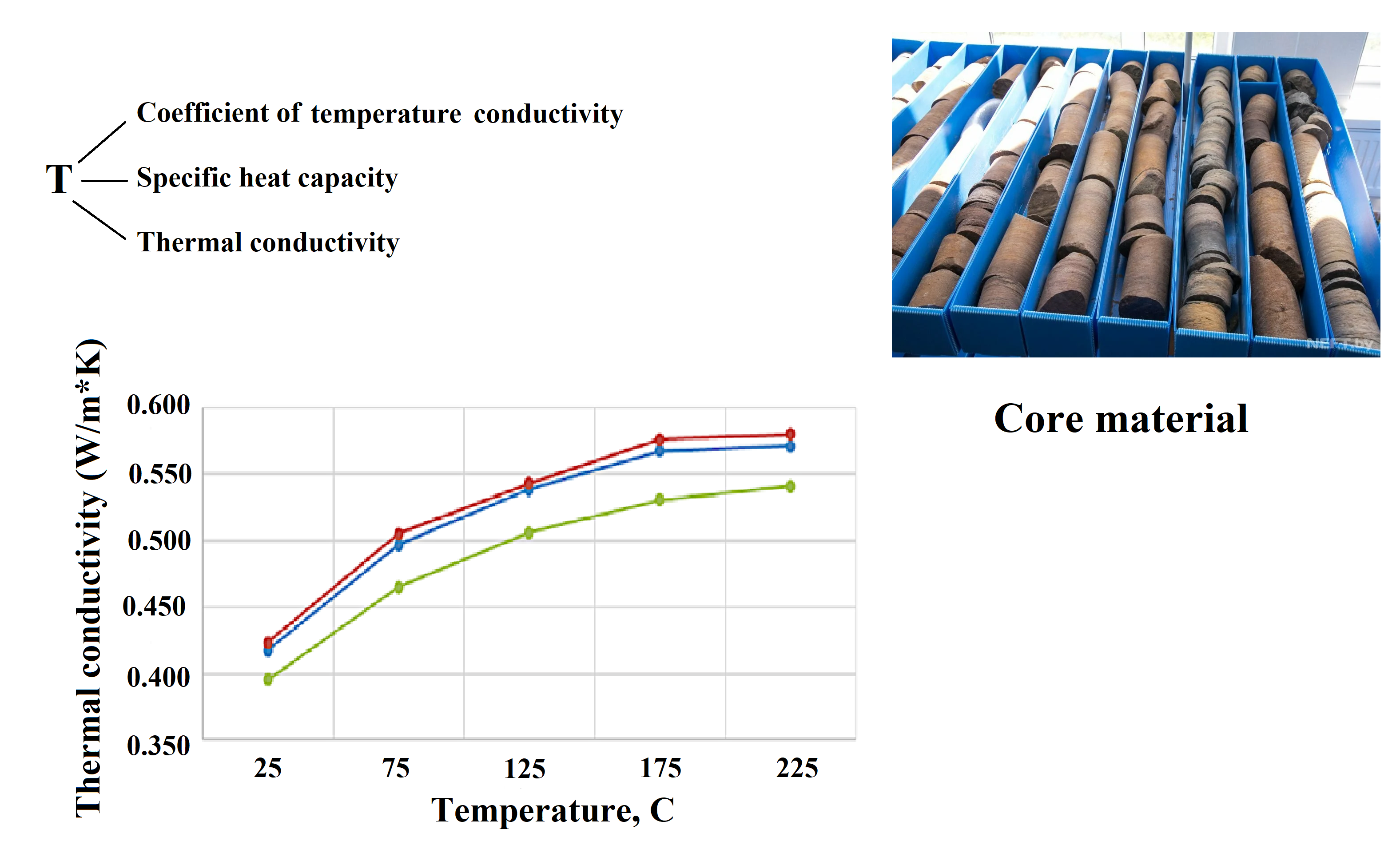The absorption of heat by rocks is accompanied by an increase in the kinetic energy of molecules and atoms and is recorded by a change in rock temperature. The thermal properties of rocks characterize the ability to transfer and absorb heat and change their size when the temperature rises. The main thermal properties of rocks are thermal conductivity, heat capacity, linear thermal expansion, and thermal volumetric expansion. In this paper, the influence of temperature effects on the thermal properties of reservoirs – the coefficient of temperature conductivity, specific heat capacity, and thermal conductivity is studied using the samples of oil-saturated reservoirs from the good number 19Bp of the Upper Uplift of Sotnikovsky deposit. The dependence of the thermal properties of the core material on temperature was revealed using a number of laboratory experiments. The results of these studies contribute to improving the reliability of data on the relationship of thermal properties with other physical properties of oil-saturated reservoirs and can be used to improve the efficiency of the development of fields of super-viscous oil.

Core material; Core material temperature; Thermal conductivity; Thermal conductivity coefficient; Specific heat capacity

Introduction

The degree of study of the thermal field of the Earth and the Earth's crust is still low, and it is incomparable with the modern results of studies of seismic, magnetic, gravitational and other physical fields of our planet. This is largely due to the insufficient level of development of thermal petrophysics (thermal physics of rocks) underlying the study of natural and artificial thermal fields in the subsurface. To solve such problems as modeling of sedimentary basins and oil and gas bearing systems, search and exploration of hydrocarbon deposits, design of thermal methods of production of high-viscosity oils, interpretation of results of thermometry in wells, determination of heat flux density from the bowels, etc. requires reliable database on thermal conductivity, thermal conductivity, the specific heat capacity of rocks (Abed and Yakhlef, 2020; Adam, 2009).

The situation is complicated by the fact that rocks, as an object of thermophysical research, have characteristic features, consideration of which is necessary when creating methods and equipment for determining their thermal properties. First, this is their essential heterogeneity, multiphase (saturation of solid rock skeleton by various pore fluids, such as formation water, gas, oil and their various combinations), dependence on thermodynamic conditions of occurrence (rock, formation pressure, temperature).

It should be noted that a significant part of the core from shallow deposits of extra-viscous oils is weakly consolidated and loose samples, which requires a special research methodology (which, until recently, was practically absent).

The variety of problems solved in mining thermal physics also determines the difference in the methods used for experimental determination of the thermal characteristics of rocks (Alas and Ali, 2019).

The choice of methods is influenced by many factors, including the purpose of the study, the range of changes in the thermal properties of rocks, different degrees of consolidation (from unconsolidated sedimentary reservoirs of ultra-viscous oils to hard low-porous rocks of the crystalline basement), the degree of sample saturation, etc. The depth of sampling determines the values of pressures at which it is necessary to study the rocks. The nature of the thermal influence determines the temperature range of research (Balzamov et al., 2020b).

The depletion of easily recoverable oil reserves leads to the need to involve in the development of increasingly complex facilities containing hard-to-recover oil reserves (HTR reserves). At the moment, the trends are that due to a decrease in the number of reserves, the lifting of traditional fossil fuels will be supplemented by the development of unconventional sources of raw materials (shale oil and gas, high-viscosity oils, bitumen, bituminous sand oil, coal methane, gas hydrates, etc.).

The world reserves of natural bitumen are estimated at more than 800 billion tons. At the same time, Russia is one of the leaders in reserves, a third of which is located on the territory of the Republic of Tatarstan.

The extraction of bituminous oil requires an unconventional, unique approach. There are various ways to develop deposits of heavy oils and natural bitumen, which differ in technological and economic characteristics.

The most promising ways are thermal methods of extracting super-viscous oil (SVO) and natural bitumen. The foundations of their application were laid by I.M. Gubkin and developed in the works of A.B. Sheinman, E.B. Chekalyuk and L.I. Rubinstein (Sheinman et al., 1969; Chekalyuk et al., 1955; Rubinstein et al., 1958). Later, applied problems in the field of thermal extraction of SVO were solved by well-known scientists from Russia, Azerbaijan, Ukraine and other countries. In this area, G.E. Malofeev, Yu. A. Zheltov, I.A. Charny, A.A. Boxerman, N.L. Rakovsky, K.A. Oganov and others made a significant contribution.
The research aims to determine the temperature dependence of the specific heat capacity and thermal conductivity of weakly cemented, bituminous-saturated sandstone samples. Data on the thermophysical properties of rocks are a key parameter for numerical models of reservoir systems. In addition, they are needed to determine the rate of advance of the coolant front, assessment of thermal resources of deposits and design development systems.

Experimental Methods

The efficiency of the lifting of SVO and natural bitumen using thermal methods largely depends on the methodological base, based on laboratory studies to determine data on the thermophysical properties of rocks (Ibragimova et al., 2017; Ismagilova et al., 2016; Valeeva et al., 2013). In addition, they are necessary for work to determine the speed of advance of the heat transfer agent front, the assessment of thermal resources of deposits and the design of development systems. All of the above determines the relevance of the topic of this work.
The LFA 467 laser burst device was used to determine the temperature conductivity. An infrared detector is used to measure the temperature increase from the back of the sample as a function of time. The measurement of temperature conductivity, specific heat capacity allows (with a known or additionally measured volumetric density) to calculate the thermal conductivity of the sample under study (Balzamov et al., 2020a; Petrov et al., 2019; Ganeeva et al., 2014).
To determine the specific heat capacity of the rock, a differential scanning calorimeter DS 204 HP was used in work. The essence of the method consists in measuring the heat of processes and the specific heat capacity of substances through the heat flux - the derivative of heat over time (differential). Heat flows are measured simultaneously by the temperature difference at two points of the measuring system (Balzamov et al., 2020c; Mirgorodskaya et al., 2018; Gabdrakhmanov et al., 2015). The determination of specific heat is carried out by a special program NETZSCH TA4_5.

The specific heat capacity is determined by the formula (1):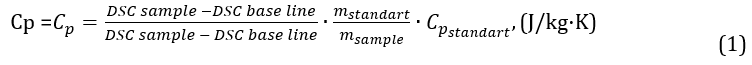Where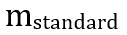is the mass of the standard;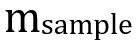is the mass of the sample.

The analytical study of thermal conductivity is reduced to the study of spatio-temporal changes in temperature and specific heat capacity, i.e., to the solution of equation (2):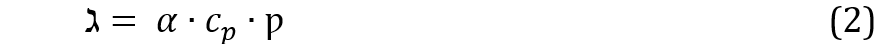where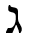is the thermal conductivity (W/ m*K); ? is the temperature conductivity (mm2/s);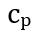is the specific heat capacity (J/kg·K); ? is the rock density = 1743 (kg/m3).

The results of this study will optimize the methods of lifting SVO. In this paper, the influence of temperature on the thermal properties of the core material is studied – the coefficient of temperature conductivity, specific heat capacity, and thermal conductivity, the purpose of which is to analyze the influence of temperature on the thermophysical properties of the core material. The temperature conductivity is measured on the LFA-467 device, and the specific heat capacity - on the DSC-204 HP device, the thermal conductivity is determined by mathematical calculation. The dependence of the thermal properties of the core material on temperature was revealed using a number of laboratory experiments. The results of these studies can be used to improve the efficiency of developing super-viscous oil fields.

The LFA 467 incorporates sophisticated equipment and simple software to provide fast, accurate and safe measurements. The LFA 467 is based on the laser flash method according to international standards ASTM E-1461, DIM EN 821 and DIN 30905.

An infrared detector measures the temperature increase on the sample's backside as a time function. The measurement of thermal diffusivity and specific heat capacity allows (with known or additionally measured bulk density) to calculate the thermal conductivity of the sample under study.

Mathematical analysis of the measured temperature dependence on time allows us to determine the thermal diffusivity ?. The analysis is performed by a special program using a set of differential mathematical models for various applications.

Under adiabatic conditions, ? is defined by the equation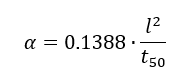Where: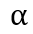– thermal conductivity (sm2/s);
l – sample thickness (sm);
t50 – time (s) corresponding to an increase in temperature by 50% (relative to the temperature at the back of the sample)

The pressure measurement holder for the LFA 467 includes a torque wrench with a torque range of 60 - 260 N×cm. The sample is positioned between the upper and lower support plates, which are placed in the cup of the holder. The pressure is increased by the locking nut (inner hexagon). In order to achieve the required compression (p) of the sample, the fixation nut must be tightened to a certain torque (M) (Figure 1).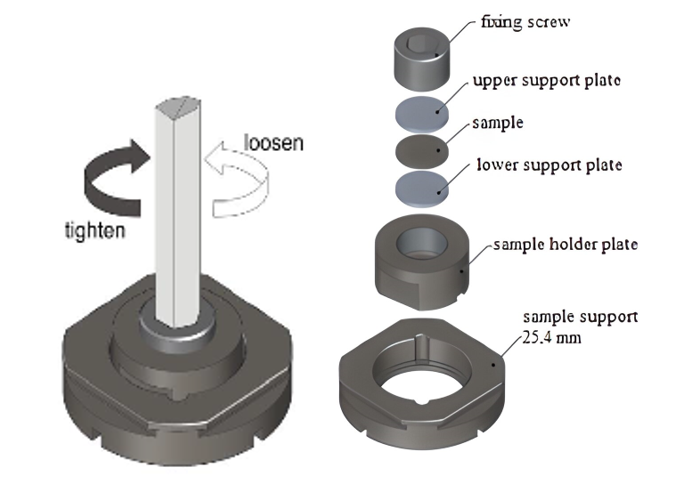Figure 1 Holder for thermal conductivity measurements

When the sample is loaded, the measuring cell LFA 467 is opened, and the samples (round or square) are placed in the corresponding sample holder. The upper flap of the furnace is put back in place with the extraction device, and the measuring cell is closed.

Start the measurement. Start the measurement by using the NETZSCH software. Determine the basic settings for the measurement. Set the gas parameters. Set the holder to be used. Mark the desired sample position in the left window (the sample will be marked green) and define the parameters of the respective sample:

- Sample properties

- Stain, layer properties

- Template for measurements

- Thermophysical properties

Temperature program. Select the start and end temperature of the measurement and determine the temperature step value (in our work, this corresponds to 50°C).

Set the required interval for analysis. The calculation interval should be approximately 10-12 half-periods.

To ensure correct estimation of the calculation interval, both curves (the original signal curve and its smoothing) should be combined (Figure 2 see on supplementary).

DS? 204 ?? - has two measuring cells: one for the test sample and one for the reference sample (Figure 3). The cells are designed as symmetrically as possible (the same crucibles, the same sensors, and the same distance from the heater to the sensor). The time dependence of the temperature difference between the sample cell and the reference cell is measured experimentally.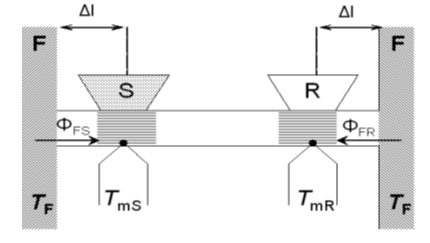Figure 3 Scheme of measurements by DSC 204 HP method

(F - furnace (heater), S - sample, R - standard, TF, TmS, TmR - temperatures of furnace and junction of differential thermocouple of sample and standard, FFS, FFR - heat fluxes)

The essence of the method consists in measuring the heat of processes and the specific heat capacity of substances through the heat flow - the derivative of heat over time (differential). Heat fluxes are measured by the temperature difference in two points of the measuring system at one point in time. Measurements are carried out both in isothermal conditions and in dynamic mode with a programmable change in the shell's temperature (heater).

Results and Discussion

Loose, highly porous (33%), fine-grained, bitumen-saturated sandstone samples of the Ufa tier of Tatarstan deposits were selected as the object of the study. Samples were taken from depths of 195.9 m, 196.0 m, and 196.1 m. The studies were carried out at temperatures of 25°C, 75°C, 125°C, 175°C, and 225°C and modeling of rock pressure corresponding to their depth of occurrence.
In a core sample taken at a depth of 195.9 m, the specific heat capacity increases from 715 to 1154 J/kg×K. As a percentage, the increase in the coefficient is 61.4% (Figure 4).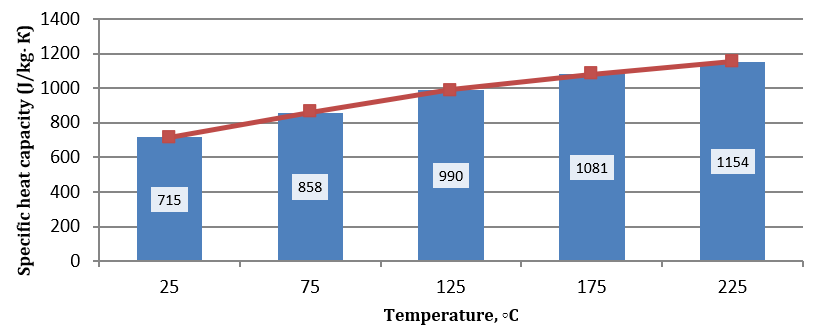Figure 4 Dynamics of changes in the coefficient of specific heat capacity at a depth of 195.9 m

At a depth of 196 m, the specific heat capacity increases from 708 to 1124 J/kg×K. As a percentage, the increase in the coefficient is 58.7% (Figure 5).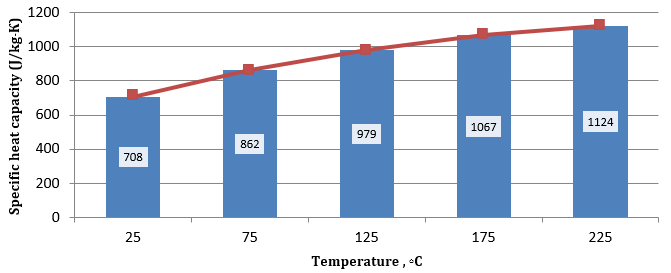Figure 5 Dynamics of changes in the coefficient of specific heat capacity at a depth of 196 m

In a core sample taken at a depth of 196.1 m, the specific heat capacity increases from 702 to 1124 J/kg×K. As a percentage, the increase in the coefficient is 60% (Figure 6).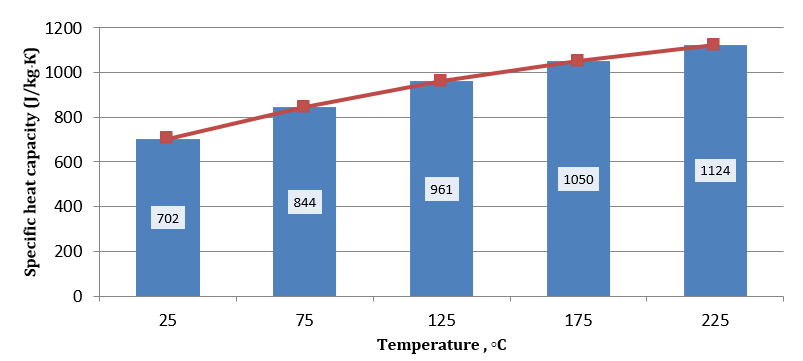Figure 6 Dynamics of changes in the coefficient of specific heat capacity at a depth of 196.1 m

According to the results of laboratory studies, the dependence of the specific heat capacity on temperature was noted. The specific heat capacity increases with an increase in the temperature of the core material (Figure 7). It should be noted that the increase in the heat capacity of the core material occurs not only with an increase in temperature but also with a change in the depth of the sample. The greater the depth of the test sample, the higher its heat capacity is.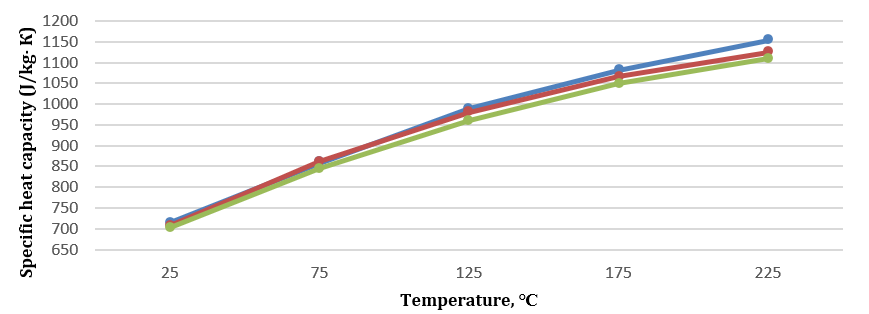Figure 7 Dependence of specific heat capacity on temperature

Where · - 195.9 m; · - 196.0 m; · - 196.1 m

Three experiments were also performed to determine the temperature conductivity of the unconsolidated core from the well of the number 19Bp of the Upper Uplift of Sotnikovsky deposit from the intervals of 195.9; 196.0; 196.1 m, at temperatures of 25?, 75?, 125?, 175?, and 225?.
In a core sample taken at a depth of 195.9 m, the thermal conductivity decreases from 0.335 to 0.284 mm2/s. The percentage reduction of the coefficient is 15.2% (Figure 8).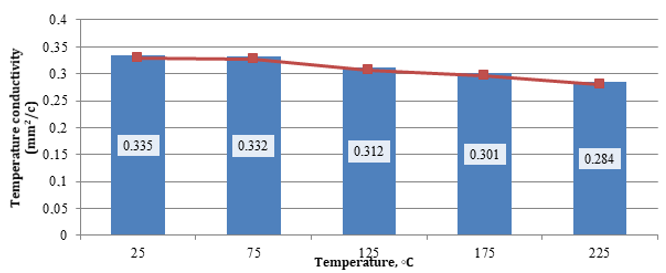Figure 8 Dynamics of changes in the temperature conductivity coefficient at a depth of 195.9 m

In a core sample taken at a depth of 196 m, the temperature conductivity decreases from 0.343 to 0.286 mm2/s. The percentage reduction of the coefficient is 16.6% (Figure 9).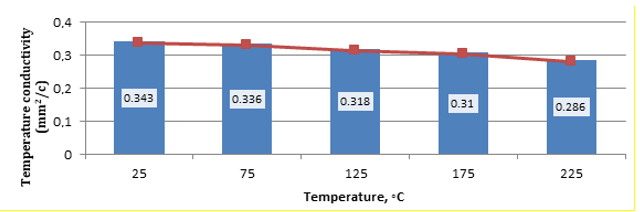Figure 9 Dynamics of changes in the temperature conductivity coefficient at a depth of 196 m

At a depth of 196.1 m, the thermal conductivity decreases from 0.323 to 0.28 mm2/s. The percentage reduction of the coefficient is 13.3% (Figure 10).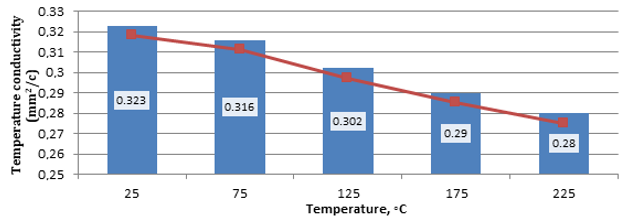Figure 10 Dynamics of changes in the temperature conductivity coefficient at a depth of 196.1 m

According to the results of the research, it was found that the coefficient of temperature conductivity decreases with an increase in the temperature of the core material (Figure 11). This is due to the fact that with an increase in the temperature of the sample, thermal expansion occurs, and the contact between the core grains worsens, as a result of which the thermal diffusivity decreases. The highest thermal diffusivity values in all research intervals are observed at a temperature of 25?. Additionally, it was noted that with an increase in bituminous content, the thermal conductivity also decreases.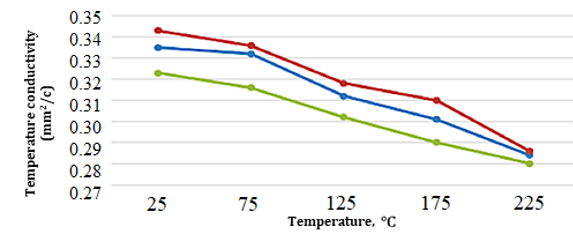Figure 11 Results of the temperature conductivity study temperature conductivity mm2/s

Where · - 195.9 m; · - 196.0 m; · - 196.1 m.

The analytical study of thermal conductivity is reduced to the study of spatio-temporal changes in temperature and specific heat capacity, i.e., to find equation 2.

When calculating the thermal conductivity according to the formula using the data of temperature conductivity and specific heat capacity, the following results were obtained (Table 1).

Table 1 Defined values of thermal conductivity of core material samples temperature

 Name of indicators T, oC Intervals of sampling of core material 195.9 m 196.0 m 196.1 m Thermal conductivity, W/m*K 25 0.417 0.423 0.395 75 0.497 0.505 0.465 125 0.538 0.543 0.506 175 0.567 0.576 0.531 225 0.571 0.580 0.541

Figures 6-8 show the direct relationship between the coefficient of thermal conductivity and

In a core sample taken at a depth of 195.9 m, the thermal conductivity increases from 0.417 to 0.571 W/m×K. As a percentage, the increase in the coefficient is 37% (Figure 12).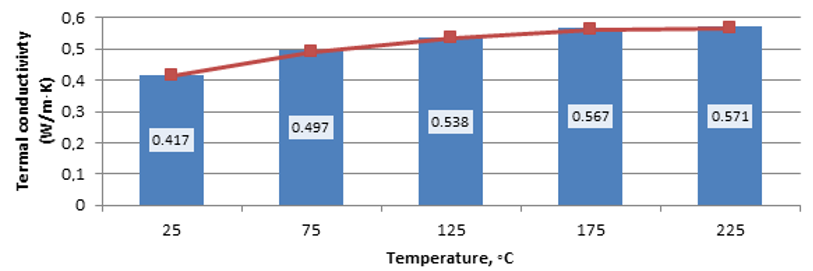Figure 12 Dynamics of changes in the thermal conductivity coefficient at a depth of 196.1 m

At a depth of 196 m, the thermal conductivity increases from 0.423 to 0.580 W/mm×K. As a percentage, the increase in the coefficient is 37.1% (Figure 13).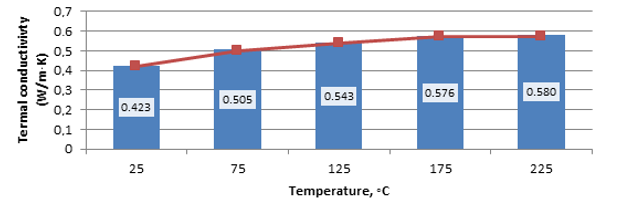Figure 13 Dynamics of changes in the thermal conductivity coefficient at a depth of 196.1 m

At a depth of 196.1 m, the thermal conductivity increases from 0.395 to 0.541 W/m×K. As a percentage, the increase in the coefficient is 36.9% (Figure 14).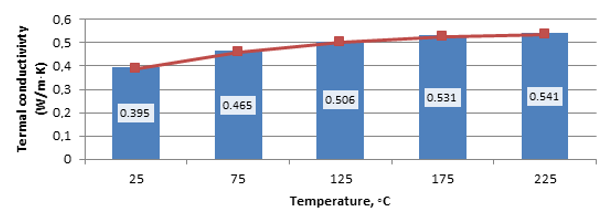Figure 14 Dynamics of changes in the thermal conductivity coefficient at a depth of 196.1 m

Based on these three intervals, it is obtained that the thermal conductivity increases with an increase in the temperature of the core material (Figure 15).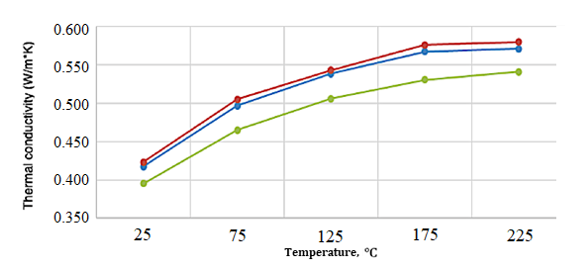Figure 15 Dependence of thermal conductivity on temperature

Where · - 195.9 m; · - 196.0 m; · - 196.1 m

The analysis showed that in the temperature range from 25°C to 225°C, depending on the depth of the core sample, the temperature conductivity varies to the greatest extent from 13.3% to 16.6% (the change is 3.3%). In this temperature range, the thermal conductivity changes to the least extent. Depending on the depth of the studied sample, the thermal conductivity varies in the range from 36.9% to 37.1% (the change is 0.2%). Depending on the depth of the sample, the heat capacity varies in the range from 58.7 to 61.4% (the change is 2.7%). With respect to thermal conductivity and temperature conductivity, the heat capacity of the core sample varies to an average degree. Depending on the depth of the sample, the heat capacity varies in the range from 58.7 to 61.4% (the change is 2.7%).
Analyzing the obtained values of heat capacity, thermal conductivity and temperature conductivity, it is possible to conclude that these parameters depend to a greater extent on the temperature of the sample, and to a lesser extent, on the depth of occurrence. So, depending on the temperature of the sample, mined from a depth of 196.0 m, its heat capacity changes by 58.8% from 708 J/kg×K at 25°C to 1124 J/kg×K at 225°C. The value of temperature conductivity with the same parameters changes by 19.93%, and thermal conductivity changes by 33.5%. Thus, when the temperature changes, the heat capacity of the core sample changes to the greatest extent.

Conclusion

According to the results of the research, it was found that the coefficient of thermal conductivity decreases with an increase in the temperature of the core material, and the coefficient of specific heat capacity increases with an increase in the temperature of the unconsolidated core. The highest values of specific heat are observed at a temperature of 225°C. The coefficient of thermal conductivity also increases with an increase in the temperature of the core material. The highest thermal conductivity values are observed at a temperature of 225°C. The increase of the thermal conductivity of the core material with the temperature change can be explained by the fact that with increasing temperature, the thermal conductivity of the medium that fills the gaps between the grains increases (the core material can be attributed to granular materials), and the heat transfer by radiation inside the granular massif also increases. The thermophysical properties of the core material obtained during laboratory studies can be applied in designing and optimizing methods for lifting viscous oil with thermal effects on the productive reservoir.

Acknowledgement

The study was carried out within the framework of a scientific project of the Russian Science Foundation (RSF) No 21-79-10406 (https://www.rscf.ru/project/21-79-10406/).

Supplementary Material
FilenameDescription
R2-CE-6009-20230330083727.docx ---

Abed, A., Yakhlef, M., 2020. Brownfield regeneration as a strategy for sustainable development: Amman case study. International Journal of Technology, Volume 11(4), pp. 732–742

Adam, R.B. 2009. Converting oil shale to liquid fuels with the Alberta Taciuk Processor: energy inputs and Greenhouse Gas Emissions. Energy & Fuels, Volume 23(12), pp. 6253– 6258

Alas, M., Ali, S.I.A., 2019. Prediction of the high-temperature performance of a geopolymer modified asphalt binder using artificial neural networks. International Journal of Technology, Volume 10(2), pp. 417– 427

Balzamov, D., Akhmetova, I., Bronskaya, V., Kharitonova, O., Balzamova, E., 2020a. optimization of thermal conditions of heat recovery boilers with regenerative heating in the high-temperature section of Isoamylene Dehydrogenation. International Journal of Technology, Volume 11(8), pp. 15981607

Balzamov, D.S., Balzamova, E.Yu., Bronskaya, V.V., Oykina G.I., Rybkina E.A.,   Shaikhetdinova, R.S., Kharitonova, O.S., 2020b. The beneficial using the heat of the exhaust gases of the furnaces of the technological unit for the ethylene oxide production. In: IOP Conference Series: Materials Science and Engineering, Volume 862(6), 062044

Balzamov, D.S., Balzamova, E.Y., Bronskaya, V.V., Rybkina, E.A., Kharitonova, O.S., 2020c. Beneficial use of thermal secondary energy resources in the rectification cycle at ethylene glycol production unit. In: IOP Conference Series: Materials Science and Engineering, Volume 919(6), 062027

Balzamov, D.S., Balzamova, E. Yu., Bronskaya, V.V., Valitov, N.V., 2020d. Possibility of using associated petroleum gas as a fuel for a production boiler house. In: IOP Conference Series: Materials Science and Engineering, Volume 791(1), 012006

Chekalyuk, E.B., 1955. The temperature field of the formation when the coolant is injected into the well. Oil economy, Volume 4, pp. 3942

Gabdrakhmanov, D.R., Valeeva, F.G., Syakaev, V.V., Lukashenko, S.S., Lakharov, S.V., Kuryashov, D.A., Bashkirtseva, N.Y., Zakharova, L.Y., Latypov, S.K., Sinyashin, O.G., 2015. Novel supramolecular system based on a cationic amphiphile bearing glucamine fragment: structural behavior and hydrophobic probe binding. Mendeleev Communications, Volume 25(3), pp. 174–176

Ganeeva, Y.M., Yusupova, T.N., Romanov, G.V., Bashkirtseva, N.Y., 2014. Phase composition of asphaltenes. Journal of Thermal Analysis and Calorimetry, Volume 115(2), pp. 1593–1600

Ibragimova, D.A., Ivanova, I.A., Sharafieva, Z.F., Kharitonova, O.S., 2017.  Evaluation of paraffin and asphaltenes phase equilibrium in petroleum systems. International Multidisciplinary Scientific GeoConference Surveying Geology and Mining Ecology Management, SGEM, Volume 17(15), pp. 147–154

Ismagilov, I.F., Kuryashov, D.A., Idrisov, A.R., Alieva, M.R, Kashapova, N.E., 2016. Supramolecular system based on cylindrical micelles of anionic surfactant and silica nanoparticles. Colloids and Surfaces A: Physicochemical and Engineering Aspects, Volume 507, pp. 255–260

Jamilatun, S., Budhijanto, Rochmadi, Yuliestyan, A., Aziz, M., Hayashi, J., Budiman, A., 2020. Catalytic Pyrolysis of Spirulina platensis Residue (SPR): Thermochemical behavior and kinetics. International Journal of Technology, Volume 11(3), pp. 522–531

Khisamov R.S., Zakirov I.S., Zakharova E.F., Bazarevskaya V.G., Abusalimova R.R., Timirov D.A., 2018. Experience of study and development of domanik deposits by example of Bavlinskoye field of Republic of Tatarstan. Petroleum Economy, Volume 11, pp. 78–83

Mirgorodskaya, A.B., Valeeva, F.G., Zakharov, S.V., Kuryashov, D.A., Bashkirtseva, N.Yu., Zakharova, L.Ya., 2018. Aggregation behavior of morpholinium surfactants in the presence of organic electrolytes. Russian Chemical Bulletin, Volume 67(2), pp. 291–296

Petrov, S., Nosova, A., Bashkirtseva, N., Fakhrutdinov, R., 2019. Features of heavy oil spraying with single evaporation. In: IOP Conference Series: Earth and Environmental Science, Volume 282(1), p. 012004

Rubinstein, L.I., 1958. About the temperature field of the formation when a hot coolant is injected into the formation: (about the articles by E.B. Chekalyuk). In: Proceedings of the Ufa Petroleum Institute, Issue 2, pp. 149173

Sheinman, A.B., Malofeev, G.E., Sergeev, A.I., 1969. The impact of heat on the reservoir during oil production. M. Nedra

Tugov A.N., Ots A., Siirde A., Sidorkin V.T., Ryabov G.A. 2016. Development of measures to improve technologies of energy recovery from gaseous wastes of oil shale processing. Thermal Engineering, Volume 63(6), pp. 430–438

Valeeva, F.G., Kuryashov, D.A., Zakharov, S.V. Vagapova, G.I., Vasilieva, E.A., Bashkirtseva, N.Yu., Zakharova, L.Ya., Konovalov, A.I., 2013. Supramolecular system 4-aza-1-hexadecyl-1-azoniabicyclo[2.2.2]octane bromide - Sodium salicylate. Aggregation and rheological properties. Russian Chemical Bulletin, Volume 62(4), pp. 989–993